Hunt, J. F., Zhang, H., Guo, Z., and Fu, F. (2013). "Cantilever beam static and dynamic response comparison with mid-point bending for thin MDF composite panels," BioRes. 8(1), 115-129.

#### Abstract

A new cantilever beam apparatus has been developed to measure static and vibrational properties of small and thin samples of wood or composite panels. The apparatus applies a known displacement to a cantilever beam, measures its static load, then releases it into its natural first mode of transverse vibration. Free vibrational tip displacements as a function of time were recorded. This paper compares the test results from the cantilever beam static bending and vibration with standard mid-point simply supported bending samples. Medium density fiberboard panels were obtained from four different commercial sources. Comparisons were made using a set of fiberboard panels with thicknesses of 8.1, 4.5, 3.7, and 2.6 mm and nominal densities of 700, 770, 780, and 830 kg/m3, respectively. Cantilever beam static modulus and dynamic modulus of elasticity linearly correlated well but were consistently higher than standard mid-point bending modulus of elasticity having linear correlations of 1.12:1 and 1.26:1, respectively. The higher strain rates of both the static and vibrating cantilever beam could be the primary reason for the slightly higher dynamic modulus values. The log decrement of the displacement was also used to calculate the damping ratio for the cantilever beam. As expected, damping ratio had a slightly decreasing slope as density increased. This paper discusses the new apparatus and initial results.

Cantilever Beam Static and Dynamic Response Comparison with Mid-Point Bending for Thin MDF Composite Panels

John F. Hunt,a,* Houjiang Zhang,b Zhiren Guo,b and Feng Fu c

A new cantilever beam apparatus has been developed to measure static and vibrational properties of small and thin samples of wood or composite panels. The apparatus applies a known displacement to a cantilever beam, measures its static load, then releases it into its natural first mode of transverse vibration. Free vibrational tip displacements as a function of time were recorded. This paper compares the test results from the cantilever beam static bending and vibration with standard mid-point simply supported bending samples. Medium density fiberboard panels were obtained from four different commercial sources. Comparisons were made using a set of fiberboard panels with thicknesses of 8.1, 4.5, 3.7, and 2.6 mm and nominal densities of 700, 770, 780, and 830 kg/m3, respectively. Cantilever beam static modulus and dynamic modulus of elasticity linearly correlated well but were consistently higher than standard mid-point bending modulus of elasticity having linear correlations of 1.12:1 and 1.26:1, respectively. The higher strain rates of both the static and vibrating cantilever beam could be the primary reason for the slightly higher dynamic modulus values. The log decrement of the displacement was also used to calculate the damping ratio for the cantilever beam. As expected, damping ratio had a slightly decreasing slope as density increased. This paper discusses the new apparatus and initial results.

Keywords: Cantilever beam; Damping ratio; Dynamic modulus; Free-vibration; Static bending; Wood composite panels

Contact information: a: USDA Forest Products Laboratory, Madison WI, 53726 USA; b: School of Technology, Beijing Forestry University, Beijing 100083, CHINA; c: Chinese Academy of Forestry, Wanshou Shan, Beijing, CHINA; *Corresponding author: jfhunt@fs.fed.us

INTRODUCTION

Evaluation of wood and wood composites properties through vibrational methods has been used with good success for several decades (Moslemi 1967; Ross and Pellerin 1994; Ilic 2003). In the literature, most studies have focused on nondestructive testing using either longitudinal stress-wave or simply supported transverse beam vibration frequency response techniques (Ross et al. 1991; Schad et al. 1995; Murphy 1997; Ross et al. 2005; Hu 2008). These studies have shown that nondestructive vibrational properties correlate well with bending and tensile moduli as well as being able to obtain damping coefficients. Much of this work focused on large structural members. As new and lighter weight composite products are being developed with increasing demands on performance, there is a need for better analysis and analytical tools to quickly differentiate products or to describe enhanced performance characteristics. Research using transverse free-vibration for wood composites has also been used and has shown similar benefits for determining E, G, and damping values (Haines et al. 1996; Yoshihara 2011). These generally use flexible supports held at the node points for free vibration. Vibration is initiated by tapping, and the resulting frequency is measured by a piezo material or microphone apparatus at one end of the beam. There is no direct correlation for displacement vs. time measurement with most of these investigations. Other equip-ment such as the dynamic mechanical analyzers (DMA) use small samples that are vibrated at a known frequency, and the vibrational response of the samples are used to measure fundamental properties of the particular beam (Kelley et al. 1987; Menard 2008; Jiang et al. 2008). This test method is very useful for exploring specific characteristics or the influence of individual parameters that can be differentiated as the samples vibrate. This test method’s primary limitation is the specimen size. DMA samples are significantly smaller and thinner than representative, as-produced, commercial samples that would be cut from typical composite panels for bending tests. According to ASTM D 1037 (ASTM 2006), bending or tensile test specimens need to be 50.8 mm wide for thickness below 6 mm and 76.2 mm wide for all other thicknesses. Specimen lengths should be nominally 24 times their thickness. This size of sample could not be used within currently manufactured DMA vibrational test equipment. Testing as-produced composite samples requires larger fixtures to measure the vibrational properties.

The USDA Forest Products Laboratory developed a dynamic cantilever beam vibration (CBV) apparatus to test thin to moderately thick as-produced wood-fiber composite materials (Turk et al. 2008). Based on the initial apparatus, the authors are working cooperatively to develop an improved cantilever beam test apparatus that measures both static bending and vibrational properties using a one test set-up. The new cantilever beam apparatus has a load cell attached at the loading point. It is possible then, during the preloading phase, to measure static load applied at a given deformation to obtain a static bending value. Then once released into its free vibration mode, direct displacement measurements are obtained, and the data can then be used to determine frequency. This apparatus has the advantage of obtaining both static and dynamic properties from the same specimen having the same test conditions with the same boundary conditions. This new apparatus reduces many test variables, resulting in improved comparisons between static and dynamic responses of a specimen. The authors understand that most vibration theory treats the static and dynamic moduli as equivalent (Harris and Piersol 2002); however, there are differences observed in the comparison of static bending and dynamic vibration data for simply supported beams (Ross et al. 1991). The goal of our research was to develop an apparatus to measure both static bending and transverse vibrational properties of cantilever beams for thin composite material analyses that uses the same specimen and test set-up for improved comparisons.

This paper discusses the equations used and preliminary test results from the new apparatus using both the static cantilever beam modulus of elasticity (SMOE) and dynamic cantilever beam vibration modulus of elasticity tests (DMOE). Comparisons were made with modulus of elasticity for standard simply supported beam (BMOE) tests. This work is part of a continuing research program to develop the cantilever beam vibra-tion apparatus for improved testing and evaluation.

Cantilever Beam Bending Equations

For static bending of a cantilever beam, as shown in Fig. 1, the equation that describes deflection is as follows,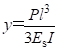(1)

where P is static load (N), y is displacement of static load point (m), l is unclamped or “free” length of the cantilever beam (m), Es is static modulus of elasticity (SMOE, Pa), and I is area moment of inertia of the beam cross section (m4).

To calculate the static MOE, we can rewrite Equation (1) in terms of known beam dimensions as follows,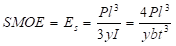(2)

where b is base width of the beam (m), and t is thickness of the beam (m).

Therefore, given a measured displacement (y) at a load (P), the SMOE can be determined. Both Equations (1) and (2) do not include shear deformation terms. It is assumed that the ratios of beams length to thickness (l:t) are so small that shear effects can be neglected. ASTM standards suggest a span length to thickness ratio of 24. For our cantilever beam, ½ the length of a full span would result in a ratio of l/t of 12. Our specimens were much longer.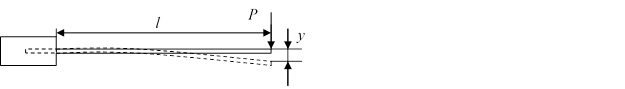Fig. 1. Static bending of a cantilever beam

Cantilever Beam Vibration Equations

The frequency of the first mode of free vibration of a cantilever beam is given by Equation (3) (Harris and Piersol 2002),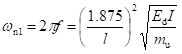(3)

where n1 is frequency of the first natural mode of vibration (radians sec–1), f is the detected frequency of the first natural mode of vibration (Hz), l is unclamped or “free” length of the cantilever beam (m), Ed is dynamic modulus of elasticity (Pa), is area moment of inertia of the beam cross section (m4), and mu is mass per-unit length (kg m–1).

Equation (3) can be rearranged and written in terms of known values to provide the dynamic modulus of elasticity (DMOE),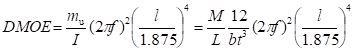(4)

where M is mass of the specimen (kg), L is total length of the specimen (m), b is base width of the specimen (m), and is thickness of the specimen (m).

Equation (4) is an idealized equation of vibration that neglects the effects of shear force and rotary motion in the specimen. Harris and Piersol (2002) calculated that if the specimen size was made such that the radius of gyration divided by the free length was less than 0.02 (dimensionless), then the frequency correction factor approaches 1.0. As the correction factor approaches 1.0, shear and rotary effects could be considered negligible for cantilever-free vibration (Eq. (5)). Thi s works out for a beam having a ratio of free length to thickness (l:t)greater than 14.5 (Eq. (6)). Then for thin composites from 1- to 10-mm thick to neglect any effects of shear, the length for the test sample length should be from 14.5 to 145 mm, respectively.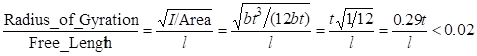(5)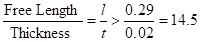(6)

Free vibration of a cantilever beam appears as a damped sine wave, as shown in Fig. 2. The damping component or the internal friction during the vibration impacts the resonant frequency so that it is less than the natural resonant frequency without damping. The logarithmic decrement of vibrational decay (δ) is a measure of internal friction and can be expressed in the form (for free vibrations) of Equation (7),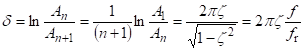(7)

where δ is the logarithmic decrement of vibrational decay, A1 is the first amplitude of the damped sine wave selected, An is the nth amplitude of the damped sine wave selected, An+1 is the (n + 1)th amplitude of the damped sine wave selected, f is the natural resonant frequency without the damping, and fr is the resonant frequency tested, damping ratio.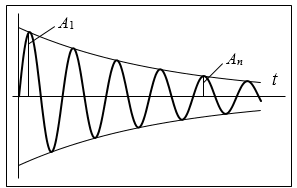From Equation (7), we can calculate the damping ratio (ζ) using the logarithmic decrement of vibrational decay (δ) in Equation (8):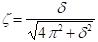(8)

Based on Equations (7) and (8), we can calculate natural resonant frequency (f) from the measured resonant frequency (fr), as shown in Equation (9).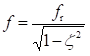(9)

The natural resonant frequency (f) can be substituted into Equation (4) to calculate the DMOE.

Simply Supported Beam Equations

The standard test method used to obtain bending MOE (BMOE) for composite panels is outlined in ASTM D1037 (ASTM 2006). Equation (10) is used to determine BMOE based on the load/deflection (∆P/∆y) curve for a simply supported beam with a constant cross section. The method suggests obtaining the linear ratio of ∆P/∆y from 10% to 40% maximum load.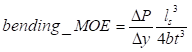(10)

where BMOE is bending modulus of elasticity (Pa), y is mid-point deflection (m), P is mid-point load (N), ls is span, simply supported beam length (m), b is base width of the specimen (m), and t is thickness of the specimen (m).

EXPERIMENTAL

Materials

Five sets of commercial medium density fiberboards (MDF) having four different fiber types, processing, thicknesses, and densities (Table 1) were tested. The materials were obtained from a local retail outlet, so the specific fiber and resin types or other manufacturing characteristics were not available for this test. One MDF panel was tested at two lengths of 340 mm and 230 mm (Sets 4 and 5). The specimen’s mass (M), total length (L), width (b), and thickness (t) were measured prior to testing. The MDF specimen size, number, and average density are listed in Table 1. The l/t ratios for the specimens ranged from 61 to 111, which was 4 to 7 times greater than the value of 14.5 that has been suggested as a minimum for including shear effects; thus, we assumed shear effects were negligible.

Cantilever Beam Apparatus and Test Methods

The cantilever beam vibration (CBV) apparatus consisted of a support base, a beam length bracket, a specimen clamp, a laser sensor, a primary displacement mechanism, and a load cell located within the displacement mechanism (Fig. 3).

Table 1. Parameters and Data for Specimens Relevant to Dynamic Testing and Mid-point Bending Testing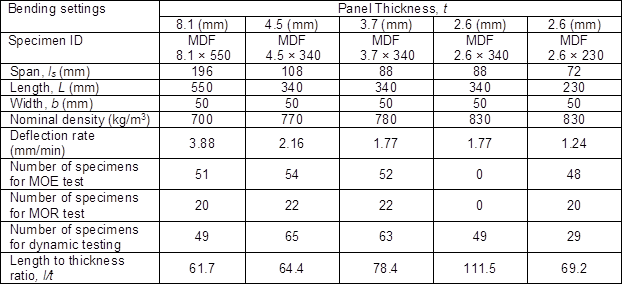The specimens were inserted 50 mm into the clamp and centered beneath a loading plate. The 50-mm grip length was subtracted from the total length (L) to obtain the free beam length (l). The specimens were clamped using a plate and screw assembly in which the screw was tightened to a constant torque to apply a constant pressure to secure the specimen. The specimen was hung vertically to minimize gravitational effects during transverse vibration. On the free end of the specimen, a laser-displacement measuring assembly was adjusted to “zero” (0) at mid-line or zero-load position. A displacement hook (not shown) was connected to a load cell and hooked to the end of the specimen to apply a consistent initial displacement of 11.1 mm. At this initial displacement, the load was recorded, and the static modulus of elasticity was calculated. The hook was released from the end of the specimen releasing the specimen to its free vibration state (first mode). The laser measured vibration displacement of the beam as a function of time. Displacement data were collected at a sampling rate of 1,000 Hz. The software determined the frequency using Equations (7), (8), and (9), then using Equation (4) to calculated the DMOE.

A typical vibration response curve for specimen (2.6 mm (t) × 50 mm (b) × 340 mm (L)) is shown in Fig. 4.

All the specimen widths were nominally 50 mm as ASTM D1037 standard specifies for specimens less than 6 mm thick. For our series, the thickest panels were (8.1 mm), which would have required a width of 76 mm. This apparatus was designed for a maximum width of 50 mm. In the future, wider clamps may be necessary; however, we chose to keep all specimen widths at 50 mm for consistent testing. The cantilever beam was initially displaced to 11.1 mm and then released into its free vibration state. The 11.1 mm initial cantilever displacement equates to approximately 30% maximum stress as calculated from modulus of rupture (MOR) (Eq. (11)) from the bending specimens.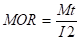(11)

where M is moment (N-m), and is area moment of inertia (m4).

Beam vibration frequencies ranged from 8.7 to 31.2 Hz resulting in 32 to 115 data points to describe each cycle within the vibration displacement curve.Fig. 3. Cantilever Beam Vibration tester shown with a specimen in position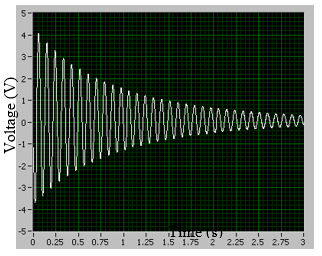Fig. 4. A typical cantilever beam free-vibration response

For mid-point testing, the span for each of the four test series was set at 24 times the nominal thickness. The respective spans for each test series are listed in Table 1. The cross-head deflection rates were set according to the ASTM test methods to provide consistent strain rates for each of the thicknesses. All of specimens were first tested using the cantilever beam vibration test, and then they were tested using the static mid-point bending test method. Approximately half of the specimens were tested to failure to obtain maximum MOR. Figure 5 shows the mid-point bending test set-up.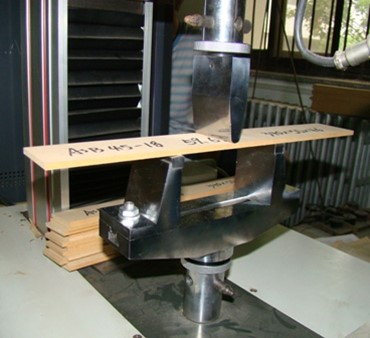Fig. 5. The mid-point bending test set-up

RESULTS AND DISCUSSION

Apparatus Repeatability

To verify repeatability of the CBV apparatus, a random specimen (2.6 mm (t) × 50 mm (b) × 340 mm (L)) was loaded and tested five consecutive times without removing it from the specimen grip or re-adjusting the positioning screws. The results show excellent repeatability, with a maximum variation in recorded frequency of 0.02 Hz (Table 2.). Similar observations were made with other samples evaluated multiple times. Also, it can be seen that the DMOE was slightly higher than the SMOE. These differences will be discussed in the next section.

Table 2. Repeated Testing Results for a Single Specimen Without Repositioning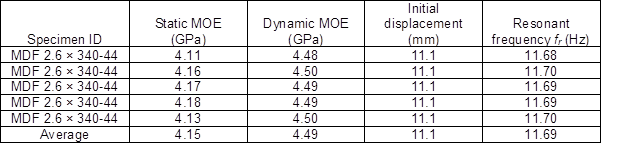Comparison of DMOE with SMOE

Figure 6 shows five plots comparing DMOE with SMOE for each of the MDF types. The DMOE had a linear correlation with SMOE for each of the board types with slopes ranging between 1.10 and 1.15. The combined average linear correlation slope was 1.12 with R2 value of 0.96 (Fig. 6(f)). This linear relationship spans the range of specimens having significantly different fiber types, processing, thicknesses, densities, and specimen length, yet the relationship is very consistent. The results show that having the same test set-up, the same test conditions, and the same specimen provided very good correlation between the two test methods.

The slightly higher DMOE values could be due to higher strain rates during beam vibration. According to ASTM D 1037, the strain rate for “static” bending should be approximately 0.005 mm/mm/min (ASTM 2006). For panels approximately 6 mm thick, ASTM D 1037 suggests using 3-mm/min cross-head movement, and for panels 12 mm thick, a rate of 6 mm/min is suggested. From the specimens tested in vibration, the highest displacement rates occurred each time the beam passed through the neutral point and slowed to zero when the beam reached maximum displacements. A conservatively low tip displacement rate estimate using total tip displacement and the beam’s natural frequency would be 4,400 to 13,200 times faster than the ASTM test method. Wood and wood composites are rate-dependent materials, and the higher the strain rates, the higher the MOE values obtained. The faster strain rate during vibration could be the significant contributor to the higher MOE values. Further analysis needs to be done to determine effects of strain rate on MOE for the CBV apparatus. The effect of higher strain rates is mentioned here, but the analysis for this effect is beyond the scope of this paper and will be addressed in later research and articles.

Comparison between DMOE, SMOE, and Mid-Point BMOE

Figure 7 shows the relationships between DMOE and BMOE for each panel series. There was good linear relationship between DMOE and BMOE on panels MDF 8.1 × 550, MDF 4.5 × 340, and MDF 2.6 × 230. The best coefficient of determination was with MDF 2.6 × 230 at 0.84. For specimens from MDF 3.7 × 340, the coefficient of determination was very low with an R2 of 0.15. This low correlation may be due to a small data spread of a single data set. When all the data were combined, the linear correlation was 1.26 with the coefficient of determination of 0.91 (Fig. 7e). Similar data were obtained (but not shown) from the static cantilever beam as compared with the standard midpoint bending test. The overall data comparison was 1.12 correlation with a 0.92 coefficient of determination (Fig. 7e). Both DMOE and SMOE showed excellent overall correlations with BMOE. However, the slightly higher DMOE and SMOE values could be partially due to higher strain rates during beam vibration, as described previously, as well as higher strain rates due to the quick application of the initial 11.1-mm displacement to the tip of the beam. Both displacement rates were faster than the 3- to 6-mm/min displacement rate used for the midpoint bending test.

Also, we realize that the clamp on the one end of the cantilever has some influence on the bending response of the beam, but we are unsure of the exact magnitude. A possible influence on the DMOE value is the effective length determination, l, of the unclamped portion of the beam. In Equation 4 the unclamped length is quadrupled, so if there were an influence, then it might show up based on differences in the free lengths used to calculate DMOE. If there were an influence of 1 mm beneath the clamp that might add to the effective length, then the calculated DMOE would be 0.8, 1.4, and 2.2 % higher for the 550 mm, 340 mm, and 230 mm long specimens, respectively. It would require an effect under the clamp of 10.7 mm to change the DMOE by 26 % for the 230 mm long specimens. The 340 mm and 550 mm beams would change by 8.6% and 15.2 % for a 10.7 mm increase in the effective length, respectively. The higher DMOE values over the BMOE as shown in Figure 7(e) are linear at about 26 % greater than the BMOE. For our set-up, we believe the clamp had only a minimal effect on the free length.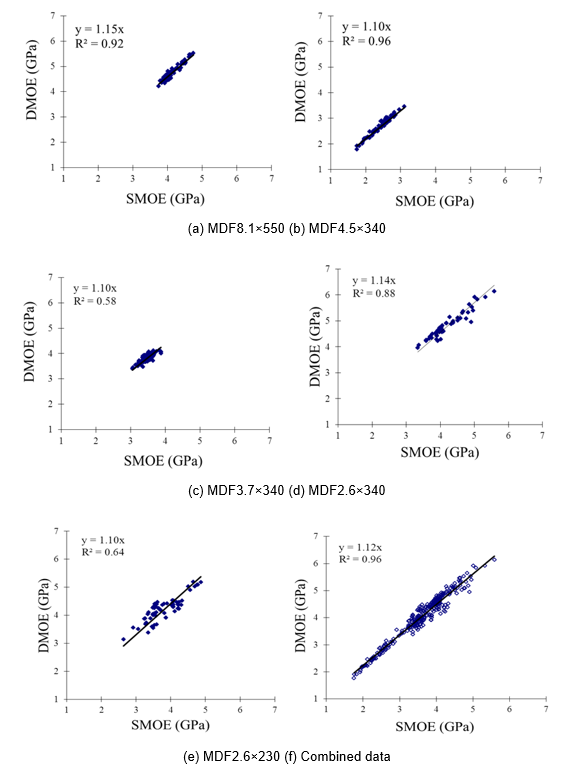Fig. 6. DMOE and SMOE relationship tested by the cantilever bending apparatus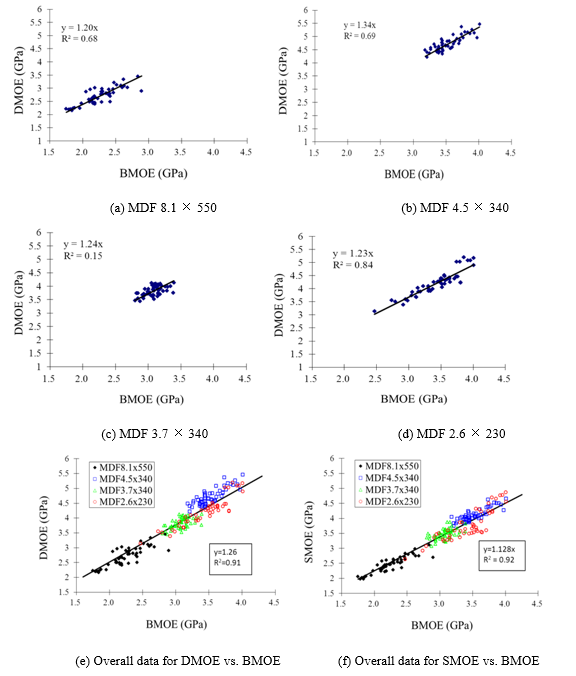Fig. 7. Relationship between DMOE, SMOE, and mid-point BMOE

We know that the pressure from the clamps decreases slightly the thickness of the beam, thus creating a thinner moment of inertia (I) for the beam at the insertion point that then should increase the deflection for a given load according to Equation (1) and as a result would decrease the SMOE. However, the SMOE was still higher than the BMOE. The boards used in this study were relatively high in density, and the decrease in thickness would be minimal. There is a need to study the exact influence of the clamps, but we believe that the effects are minimal.

Another factor that may have influenced the bending difference between the DMOE and BMOE may have come from the higher shear strain or deflection that would result in a lower calculated BMOE. The span was 24 times the thickness according to ASTM standards, but there may have been sufficient shear to lower the BMOE values. There is a need to further examine the comparison based on shear strain influences of the mid-span, static cantilever bend test, and the cantilever beam vibration test.

The authors also understand that moisture content has a strong influence on material properties. For this test sequence, there was time between testing for the DMOE and BMOE. Moisture control was not possible for this test sequence. Therefore there may have been slight property differences (up or down) due to moisture content fluctuation that would then have influenced the mechanical properties obtained from either the DMOE or BMOE testing. However, for the DMOE and SMOE testing, there would be no time difference because same specimen was used for both tests and would have been tested at the same time with the same set-up, thus eliminating any moisture content influences for their comparison.

Damping Ratio and DMOE Relationship with Density

The damping ratio (ζ) for all specimens ranged between 0.06 and 0.12 (Fig. 8). As expected, damping decreased as density increased. Damping ratio relates to the lost energy as stress is transferred within the board. Since increased density generally implies improved bonding (between fibers), it suggests better fiber–network connections and lower energy losses during vibration. The relationship between damping ratio and density was plotted as a linear relationship. However, damping ratio (energy loss) is more complex than a linear relationship and is affected by many interacting parameters other than by average density alone, such as density distribution through the thickness. In Fig. 9, MDF 4.5 × 340 samples (circled) showed higher DMOE than the others, but the plot of the damping ratio vs. density (Fig. 8.) shows no significant differences compared with the other panels. The differences may be a combined effect from density profile, fiber alignment, fiber type, resin amount, or resin type for the MDF 4.5 series.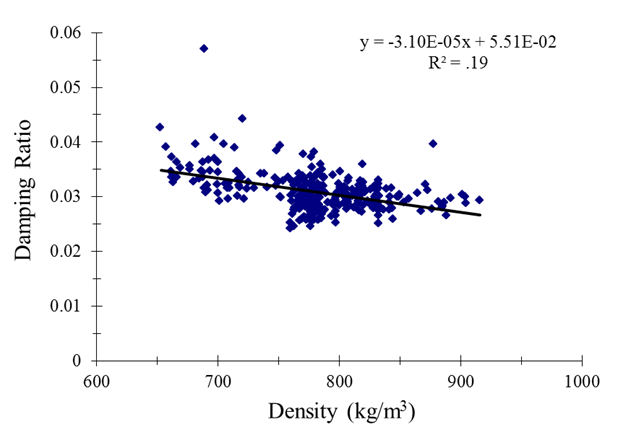Fig. 8. Relationship for Damping Ratio as a function of density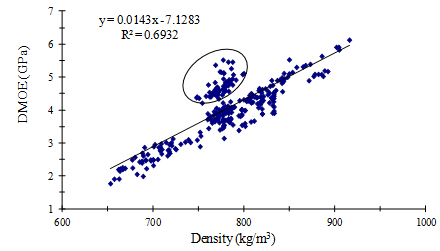Fig. 9. DMOE as a function of density. Circled data are samples from MDF 4.5 × 340 panel

CONCLUSIONS

As composites become more complex and are required to achieve improved performance, there is a need to measure and study performance differences such that the panel can be engineered for a particular performance criterion. Additional study will be required to sort out the influences of other panel parameters on the interaction between static and dynamic properties.

1. The cantilever beam apparatus provides an easy method to measure pre-load and end displacement of a fiberboard composite beam that can then be used to determine static beam mechanical properties. The SMOE of MDF beams correlates very well with mid-point BMOE.
2. The cantilever beam apparatus provides an easy method to initiate a free vibration of a beam and measure end displacement as a function of time. Tip displacement vs. time can be used to determine frequency. Then from the physical properties of the beam, the DMOE of the beam can be determined. Overall DMOE of MDF beams correlate very well with mid-point BMOE.
3. Damping ratio was shown to decrease as density increased. However, the relationship is more complex than a simple linear correlation with density.
4. The testing is nondestructive and highly repeatable for determining SMOE, DMOE, and damping. With one test set-up, the CBV apparatus allows multiple measurements that can provide more complex analyses and may provide better understanding of the composite panel than could be obtained with just one static test.
5. The cantilever beam can be cut from as-produced composite panel pieces to determine vibrational properties.
6. Additional research is necessary to determine reasons for the difference between test methods for determining MOE.
7. Additional research is necessary to determine how other panel properties such as density profile through the thickness, fiber alignment, and fiber-type impact cantilever static and dynamic properties.

Although the CBV apparatus provides more information than the standard bending test method, both the USDA Forest Products Laboratory and Beijing Forestry University will continue to cooperatively develop the cantilever beam apparatus. Our research has shown that the apparatus has the potential to provide qualitative and quantitative information that can be used to study and understand the fundamental material properties of as-produced thin fiber-based composites. Additional research is necessary to determine effects of strain rate, clamping length, clamping pressure, width of sample, density profile, fiber length, and fiber orientation on the static and dynamic moduli values.

ACKNOWLEDGMENTS

The authors thank the 948 fund of Chinese State Forestry Administration for funding this research, grant #: 2008-4-69. The corresponding author thanks C. Turk and D. J. Marr from USDA Forest Products Laboratory for their initial CBV development.

REFERENCES CITED

ASTM (2006). Standard D 1037. “Evaluating the properties of wood-base fiber and particle panel materials,” American Society for Testing and Materials, West Conshohocken, PA.

Haines, D. W., Leban, J. M., and Herbe, C. (1996). “Determination of Young’s modulus for spruce, fir and isotropic materials by the resonance flexure method with comparisons to static flexure and other dynamic methods,” Wood Sci. and Tech. 30, 253-263.

Harris, C. M., Piersol, A. G. (2002). Shock and Vibration Handbook, 5th Ed. McGraw-Hill, New York, 2002, 1568 pp.

Hu, Y.C. (2008). “Nondestructive testing of mechanical parameters for wood-based materials,” Proceedings: 17th World Conference on Nondestructive Testing, 25–28 Oct 2008, Shanghai, China.

Ilic, J. (2003). “Dynamic MOE of 55 species using small wood beams,” Holz Roh Werkst. 61(3), 167-172.

Jiang, J. L., Lu, J. X., and Yan, H. P. (2008). “Dynamic viscoelastic properties of wood treated by three drying methods measured at high-temperature range,” J. Wood Fiber Sci. 40(1), 72-79.

Kelley, S. S., Rials, T. G., and Glasser, W. G. (1987). “Relaxation behavior of the amorphous components of wood,” J. Mater. Sci. 22(2), 617-624.

Menard, K. P. (2008). Dynamic Mechanical Analysis: A Practical Introduction, CRC Press, Boca Raton, FL.

Moslemi (1967). “Dynamic viscoelasticity of hardboard,” Forest Prod. J. 17(1), 25-33.

Murphy, J. F. (1997). “Transverse vibration of a simply supported beam with symmetric overhang of arbitrary length,” J. Test. Eval. 25(5), 522-524.

Ross, R. J., Geske, E. A., Larson, G. L., and Murphy, J. F. (1991). “Transverse vibration nondestructive testing using a personal computer,” Res. Pap. FPL–RP–502. USDA, Forest Products Laboratory, Madison, WI.

Ross, R. J., and Pellerin, R. F. (1994). “Nondestructive testing for assessing wood members in structures: A review,” FPL–GTR–70. USDA, Forest Products Laboratory, Madison, WI.

Ross, R. J., Zerbe, J. I., Wang, X., Green, D. W., and Pellerin, R. F. (2005). “Stress wave nondestructive evaluation of Douglas-Fir peeler cores,” Forest Prod. J. 55(3), 90-94.

Schad, K. C., Kretschmann, D. E., McDonald, K. A., Ross, R. J., and Green, D. A. (1995). “Stress wave techniques for determining quality of dimensional lumber from switch ties,” FPL–RN–0265. USDA, Forest Products Laboratory, Madison, WI.

Turk, C., Hunt, J. F., and Marr, D. J. (2008). “Cantilever-beam dynamic modulus for wood composite products: part 1 apparatus,” FPL–RN–0308. USDA, Forest Products Laboratory, Madison, WI.

Yoshihara, H. (2011). “Measurement of the Young’s modulus and shear modulus of in-plane quasi-isotropic medium-density fiberboard by flexural vibration,” BioResources 6, 4871-4885.

Article submitted: August 16, 2012; Peer review completed: September 29, 2012; Revised version received and accepted: November 1, 2012; Published: November 12, 2012.